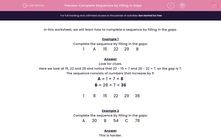# Complete Sequences by Filling in Gaps

In this worksheet, students must fill in the gaps in the given number sequences.This content is premium and exclusive to EdPlace subscribers.Key stage:  KS 2

Curriculum topic:   Maths and Numerical Reasoning

Curriculum subtopic:   Place Value

Difficulty level:#### Worksheet Overview

In this worksheet, we will learn how to complete a sequence by filling in the gaps.

Example 1

Complete the sequence by filling in the gaps:

 1 A 15 22 29 B

Look for clues.

Here we look at 15, 22 and 29 and notice that 22 - 15 = 7 and 29 - 22 = 7, so the gap is 7.

The sequence consists of numbers that increase by 7.

A = 1 + 7 = 8

B = 29 + 7 = 36

 1 8 15 22 29 36

Example 2

Complete the sequence by filling in the gaps:

 A 30 B 54 C 78

This is harder.

54 - 30 = 24

78 - 54 = 24 also.

The gap will be half this, i.e. 12.

A = 30 - 12 = 18

B = 30 + 12 = 42

C = 54 + 12 = 66

 18 30 42 54 66 78

### What is EdPlace?

We're your National Curriculum aligned online education content provider helping each child succeed in English, maths and science from year 1 to GCSE. With an EdPlace account you’ll be able to track and measure progress, helping each child achieve their best. We build confidence and attainment by personalising each child’s learning at a level that suits them.

Get started••••